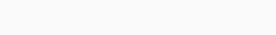# Explain R Squared used In Machine Learning in Python

In simple words, R Square is a statistical formula. Here we get more details.

## What is R square?

R2  is just a square of R. R is a correlation that is numbered between +1 and -1. It shows the relationship between the dependent variable and the independent variables. Values close to  +1 or -1 shows a strong relationship between both variables. If value tends to 0 it shows the variable is less likely to depend on each other. R2 is only shown variation from 0 to 1. It does not show the direction(increase or decrease) of a variable. We are using R2  because it’s interpretation is very easy. The calculation is also easy in R2. Higher the R2  value than the higher the information in variables.

## R square in machine learning in Python

Here, we implement the R square in machine learning using a house price dataset.

We importing libraries. We are using python library Numpy, Pandas, Sklearn, Scipy.

```#imporing libraries
import numpy as np
import pandas as pd
import matplotlib.pyplot as plt
from sklearn.metrics import r2_score
from sklearn.linear_model import LinearRegression
from scipy import stats```

Now, we preparing our data. Here, the House price dataset is used.

```#Praparing Data
data=data.sort_values(["price"],ascending=True)
data=data[['price','sqft_lot']][:10]

Output:-Plotting our data:

```#plotting the data
X=np.array(data['price']).reshape(-1,1)
Y=np.array(data['sqft_lot'])
plt.scatter(X,Y)```

Output:-Now, we call function LinearRegression() and fits data into it and predicting Y value for value X. and then plotting the data with linear regression.

```#performing linear regression
LR=LinearRegression()
LR.fit(X,Y)
y_prediction=LR.predict(X)```
```#plotting linear Regression
plt.scatter(X,Y)
plt.plot(X,y_prediction,color='green')```

Output:-Creating a function for calculating variance. A variance of the mean calculated by all value subtracted by it’s mean and finding the sum of all numbers.

var(mean)=sum(Y-mean)2

```#function for variaton
def var(Y1,Y2):
var=sum((Y1-Y2)*(Y1-Y2))
return var```

Now, creating a function for calculating R2. The formula for calculating R2 is as follows:

R2 = (var(mean) – var(line))/var(mean) = 1-(var(line)/var(mean))

```#function for clculating R squared
def R_squared(y,y_prec):
y_mean=[y.mean() for i in y]
R_square=1-(var(y,y_prec)/var(y,y_mean))
return R_square```
```R2=R_squared(Y,y_prediction)
print("R square: ",R2)```

Output:-